Latest Banking jobs   »

# Quantitative Aptitude Quiz For SBI Clerk Mains 2022- 26th December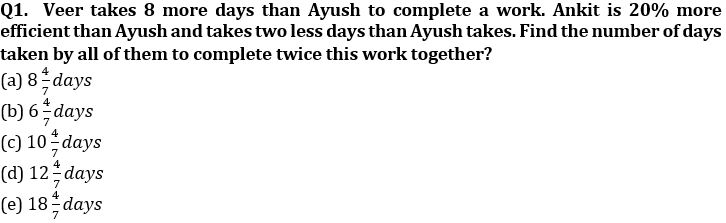Q2. Distance between two cities A & B is 600 km. P starts from A and Q from B at same time. After two hours, P realized he was travelling slow and therefore increased his speed by ¼ th and meet Q at a point 216 km from A. Find the increased speed of P, if Q drove at a constant speed of 150 km/hr.
(a) 125 km/hr
(b) 120 km/hr
(c) 80 km/hr
(d) 100 km/hr
(e) None of these

Q3. A cruise ship X is 380 m long and travels at a speed of 32 kmph in still water. Another cruise ship Y which is 180 m long travels at 40 kmph in still water. The two ships pass each other traveling in opposite directions, in a region where the speed of current is 8m/s. How long (in seconds) will it take them to pass each other?
(a) 42 sec
(b) 28 sec
(c) 35 sec
(d) 24 sec
(e) 21 sec

Q4. A basket contains only three kinds of fruits – guavas, mangoes and pears. What is the minimum number of fruits that should be picked from the basket to ensure that at least 5 guavas or at least 7 mangoes or at least 8 pears are picked?
(a) 20
(b) 19
(c) 17
(d) 18
(e) can’t be determined

Q5. A trader gives p% discount on the marked price and makes (p + 25) % profit. If he offers a discount of (p+10) %, then he makes (p+10) % profit. Find the percentage by which the marked price exceeds the cost price.?
(a) 40%
(b) 50%
(c) 45%
(d) 30%
(e) 60%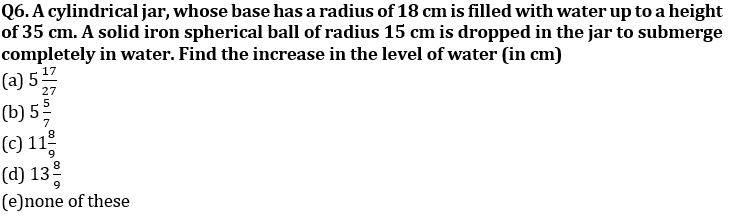Q7. An article is sold at 30% profit. Had it been sold at Rs. 155 more than previous selling price and the cost price were also increased by Rs. 100 then profit percent would have been 5% more. Find CP of the article.
(a) Rs. 500
(b) Rs. 400
(c) Rs. 460
(d) Rs. 480
(e)none of these

Q8. Anand marks up the price of an article by 50% and then allows a discount of 20% and sells it to Balaji. Balaji sells it for Rs. 20 more than what he purchased for, this S.P is 30% more than the original C.P. of the article. Then Balaji’s profit% is (rounded off to two decimal places).
(a) 7.5%
(b) 6.66%
(c) 8.34%
(d) 9%
(e) 10.25%

Q9. Four persons started to do a work together. ‘A’ works only in starting two days after that B, C and D works alternately starting from B. Ratio of time taken by A, B, C and D, if they work alone is 4 : 3 : 2 : 5. If the work is completed in 12 days then in how many days A and C can complete the work if they work together ?
(a) 6 days
(b) 12 days
(c) 10 days
(d) 8 days
(e) None of these

Q10. 2n years ago, the age of Raju was four times that of his son and n years ago, the age of Raju was thrice that of his son. If n years later, the sum of the ages of Raju and his son will be 80 years, then the difference in the ages of Raju and his son is
(a) 20 years
(b) 40 years
(c) 24 years
(d) 30 years
(e) 35 years

Q11. There are two vessels A and B which contains mixture of sulphuric acid and nitrous oxide in the ratio of 7 : 2 and 3 : 4 respectively. Mixture of both vessels are mixed to obtain a mixture of 390 ml, in which quantity of nitrous oxide is 160 ml. Find ratio of quantity of mixture in vessel A quantity of mixture in vessel B?
(a) 7 : 6
(b) 6 : 7
(c) 5 : 7
(d) 7 : 9
(e) 4 : 7

Q12. The surface area of a sphere is 423.5 cm² less than total surface area of a hemisphere. If ratio between radius of hemisphere and sphere is 3 : 2, then find the radius of hemisphere?
(a) 5.5 cm
(b) 5 cm
(c) 4 cm
(d) 7 cm
(e) 10.5 cm

Q13. A 240 meters long train crosses a 210 meters long train running in opposite direction in 6 sec. Ratio between speed of longer train and smaller train is 7 : 8. If faster train crosses a platform in 9 sec, then find time taken by slower train to cross a bridge, which is 60 meters more long than platform?
(a) 142/7 sec
(b) 136/7 sec
(c) 90/7 sec
(d) 148/7 sec
(e) 142/7 sec

Q14. Raman invested some amount in scheme ‘P’ which offer 20% C.I. p.a while some amount in scheme ‘Q’ which offers 8% S.I. p.a. After 2 year ratio of interest, earn from ‘P’ to ‘Q’ is 11 : 6. Amount invested by Raman in scheme ‘P’ is what percent of the amount invested by him in scheme ‘Q’.
(a) 50%
(b) 33⅓%
(c) 25%
(d) 66⅔ %
(e) 150%

Q15. A, B and C entered into a partnership business, A invested Rs. x, B invested 25% more than A and C invested 20% more than B for first 6 months. After that A left the business and B withdraw 50% of his investment. B left the business after 3 more months whereas C increased his investment by 16⅔% . If at the end of year difference between profit share of C and (A + B) together is Rs. 12375, then find profit share of C ?
(a) 58500 Rs.
(b) 56500 Rs.
(c) 55680 Rs
(d) 55580 Rs.
(e) 52680 Rs.

Solutions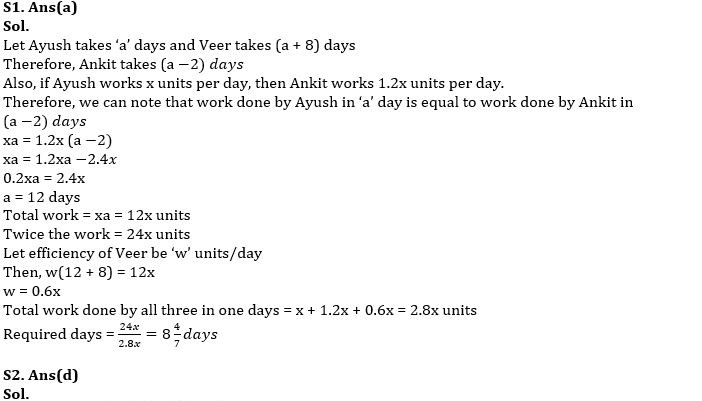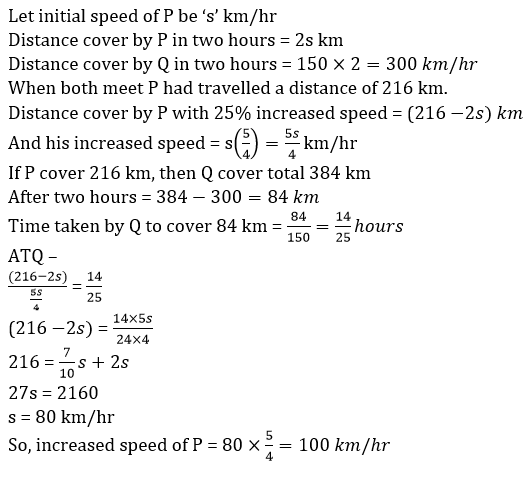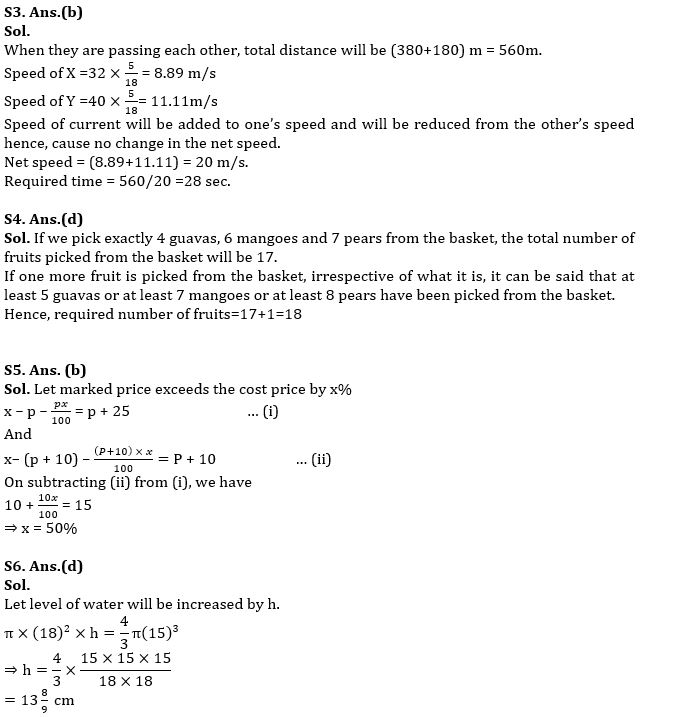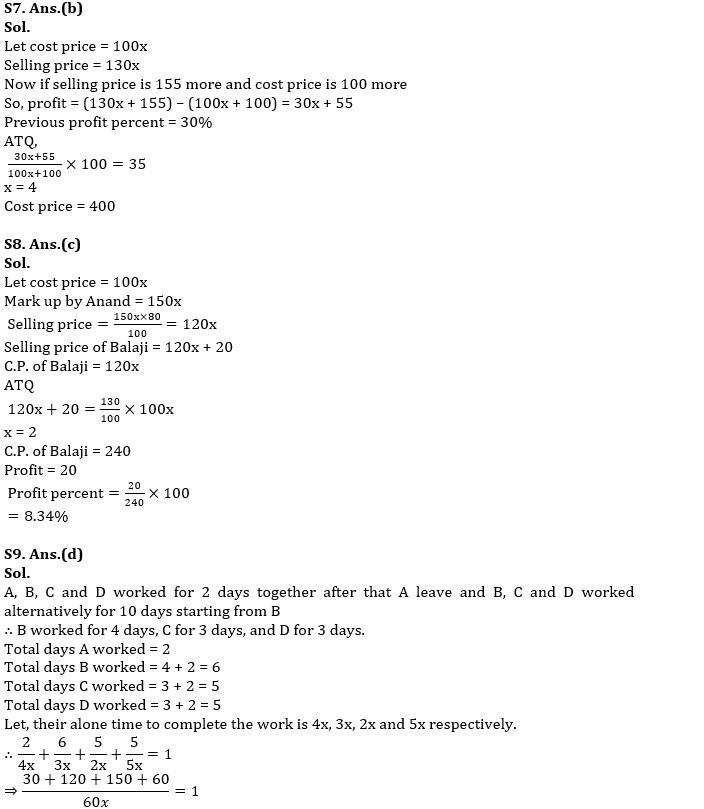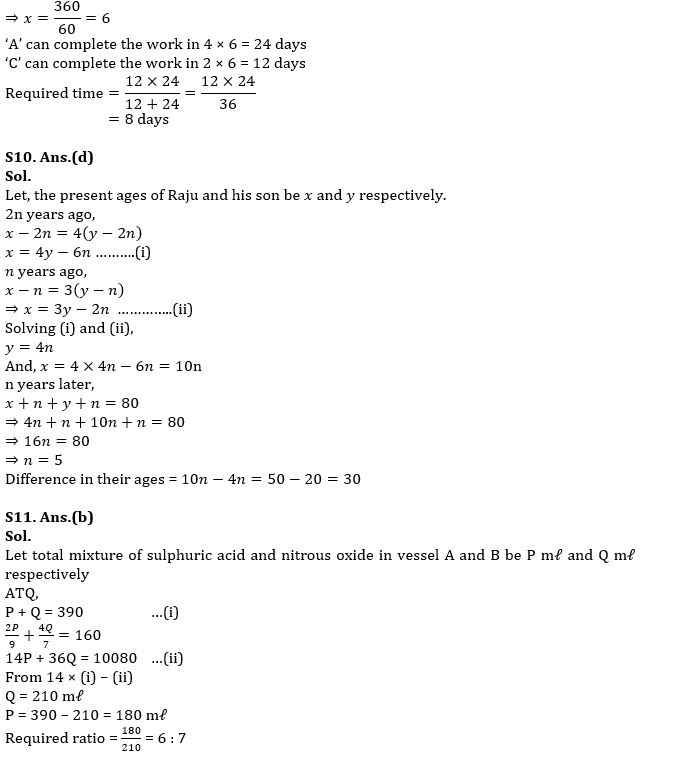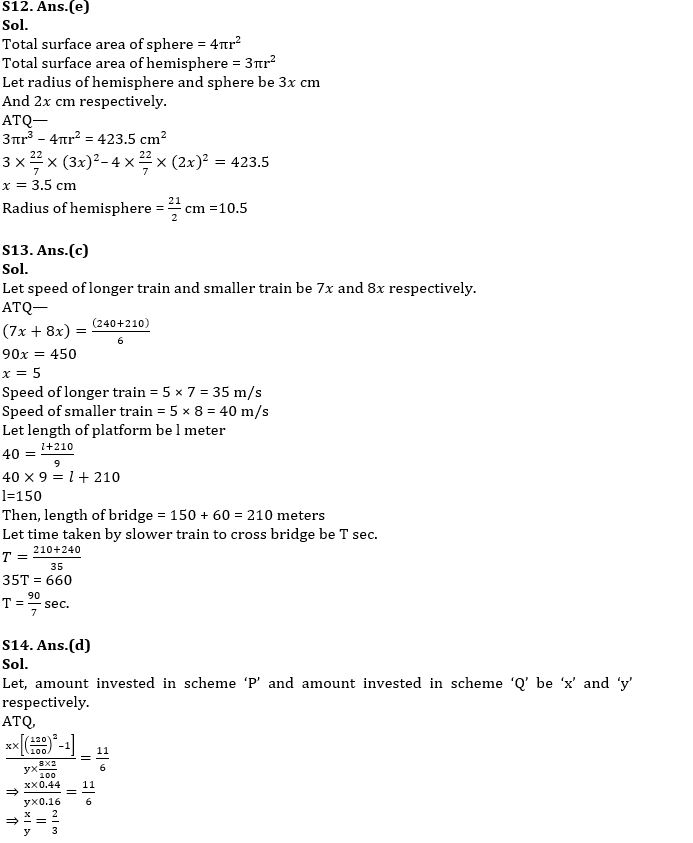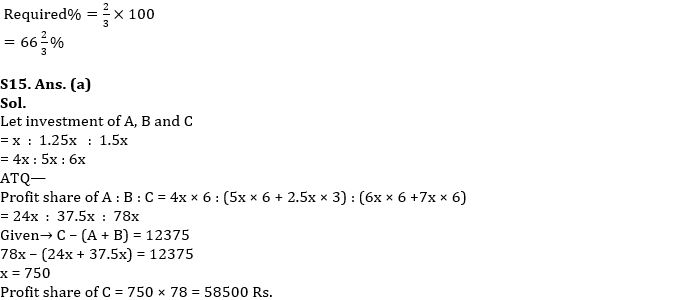#### Congratulations!Union Budget 2023-24: Free PDF